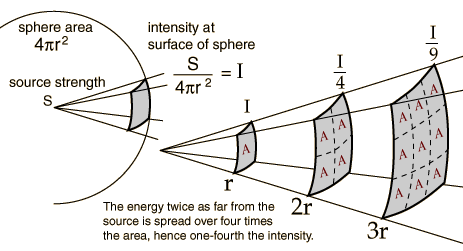# Square Law

Fundamental to Russell's energy accumulation syntropy and dispersion entropy concept is the Square Law and Inverse Square Law. Accumulating energy from a 3D VOLUME of space is a geometric consideration as the volume is reduced in successive stages or steps (tones). Frequency on the other hand is an arithmetic progression or regression as it divides or multiples 2D lengths or times. See Part 12 - Russells Locked Potentials for greater detail.

Square Law (Radio) - Contrary to Ohm's Law as voltage is increased, resistance is decreased. Ghiraldi, Alfred A.; Radio Physics Course, pg 497

Inverse Square Law Any point source which spreads its influence equally in all directions without a limit to its range will obey the square law (entropy). This comes from strictly geometrical considerations. The intensity of the influence at any given radius r is the source strength divided by the area of the sphere. Being strictly geometric in its origin, the inverse square law applies to diverse phenomena. Point sources of gravitational force, electric field, light, sound or radiation (entropy) obey the inverse square law.

The energy twice as far from the source is spread over four times the area, hence one-fourth the intensity. Gravity, electricity, sound, light and radiation obey the Inverse Square Law.

Acoustics Under far field/free field conditions, sound intensity varies inversely with the square of the distance from the source. The difference in Sound Pressure level between far field locations is expressed as:

Lp2 = Lp1 - 20 log(R2/R1)

where: Lp1 = Sound Pressure level in decibels at location 1 Lp2 = Sound Pressure level in decibels at location 2 R1 = distance from noise source to point 1 R2 = distance from noise source to point 2 (R1 and R2 must have the same units)(image courtesy of HyperPhysics

Square-Cube Law The square-cube law (or cube-square law) is a principle, drawn from the mathematics of proportion, that is applied in engineering and biomechanics. It was first demonstrated in 1638 in Galileo's Two New Sciences. It states:

"When an object undergoes a proportional increase in size, its new volume is proportional to the cube of the multiplier and its new surface area is proportional to the square of the multiplier."